Handbags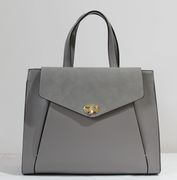MODEL NUMBER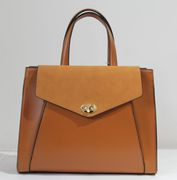MODEL NUMBER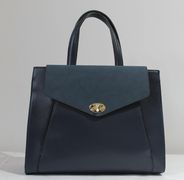MODEL NUMBER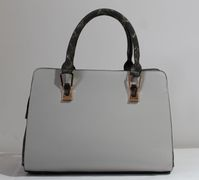MODEL NUMBER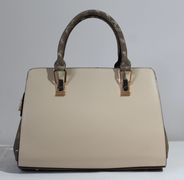MODEL NUMBER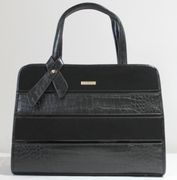MODEL NUMBER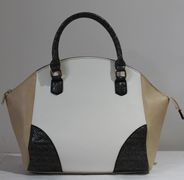MODEL NUMBERMODEL NUMBERMODEL NUMBER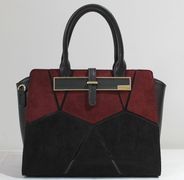MODEL NUMBER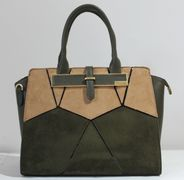MODEL NUMBER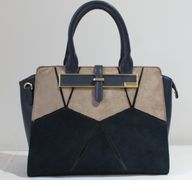MODEL NUMBER